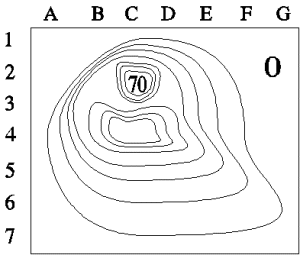Teaching Physics with the Physics Suite Edward F. Redish Problems Sorted by Type | Problems Sorted by Subject | Problems Sorted by Chapter in UP

Orienteering an electric potential

 1. (a) The figure on the right shows a contour plot of a piece of a range of hills in Virginia. The outer part of the figure is at sea level (marked 0). Each contour line from the region marked zero shows a level 10 m higher than the previous. The maximum height is 70 m and is shown by the number 70. Answer the following questions by giving the pair of grid markers (a letter and a number) closest to the point being requested. Where is there a steep cliff? Where is there a pass between two hills? Where is the easiest climb up the hill? (b) Now suppose the figure represents instead a plot of the electric equipotentials for the surface of a glass plate. The numbers now represent voltage. The maximum is 70 V and each contour line from the region marked zero shows a level 10 V higher than the previous. Where would a test charge placed on the glass feel the strongest electric force? In what direction would it point? Is there a place on the glass where a charge could be placed so it feels no electric force? Where?Not finding what you wanted? Check the Site Map for more information.